Next: No Selection Up: Standard Runs Previous: Standard Runs

### Fitness is Necessary for Bloat -- Price's Theorem Applied to Representation Length

Price's Covariance and Selection Theorem  from population genetics relates the expected change in frequency of a gene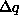in a population from one generation to the next, to the covariance of the gene's frequency in the original population with the number of offspring z produced by individuals in that population (see Equation 1). We have used it to help explain the evolution of the number of copies of functions and terminals in GP populations [15,19].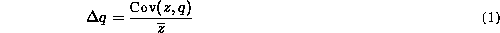In our GP the size of the population does not change so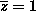and the expected number of children is given by the parent's rank so in large populations the expected change is approximately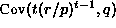as long as crossover is random. (t is the tournament size and r is each program's rank within the population of size p).

Where representation length is inherited, such as in GP and other search techniques, Equation 1 should hold for representation length. More formally Price's theorem applies (provided length and genetic operators are uncorrelated) since representation length is a measurement function of the genotype [20, page 28,]. If it held exactly a plot of covariance vs. change in mean length would be a straight line (assuming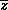is constant). The solid line plot in Figure 3 shows good agreement between theory and measurement until generation 20. After Generation 20 the rise in program length is smaller than predicted by Equation 1. The bulk of the discrepancy is due to the restriction on program length, as can be seen from the dotted line in Figure 3.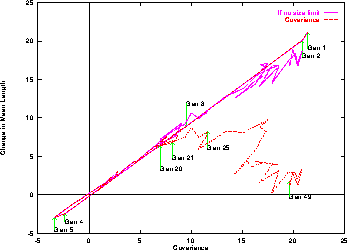Figure 3:   Covariance of program length and normalised rank based fitness v.\ change in mean length in next generation. Means of 50 runs.

Essentially Equation 1 gives a quantitative measurement of the way genetic algorithms (GAs) search. If some aspect of the genetic material is positively correlated with fitness then, other things being equal, the next generation's population will on average contain more of it. If it is negative, then the GA will tend to reduce it in the next generation.

For a given distribution of representation lengths Equation 1 says the change in mean representation length will be linearly related to the selection pressure. This provides some theoretical justification for the claim [2, page 112,] that ``average growth in size ... is proportional to selection pressure''. Which is based upon experimental measurements with a small number of radically different selection and crossover operators on Tackett's ``Royal Road'' problem . (Solutions to the Royal Road problem are required to have prespecified syntactic properties which mean ``that the optimal solution has a fixed size and does not admit extraneous code selections''. Such solutions are not executable programs.)

Referring back to Figure 2 and considering the length of the best solution and the covariance in the first four generations. We see the covariance correctly predicts the mean length of programs will increase. In contrast if we assumed the GA would converge towards the individual with the best fitness in the population, the fact that the mean length is higher than the mean length of the best individual would lead us to predict a fall in mean program length. That is Price's Theorem is the better predictor. This is because it considers the whole population rather than just one (albeit the best in the population).

Between generations 4 and 20 the mean program size can be reasonably described by a parabola, i.e. growth is quadratic. This corresponds with the covariance rising linearly (slope 0.76) with number of generations.

Where fitness selection is not used (as in the following sections), each individual in the population has the same fitness and so the covariance is always zero. Therefore Price's Theorem predicts on average there will be no change in length.Next: No Selection Up: Standard Runs Previous: Standard Runs

William B Langdon
Tue Jun 10 12:12:55 BST 1997